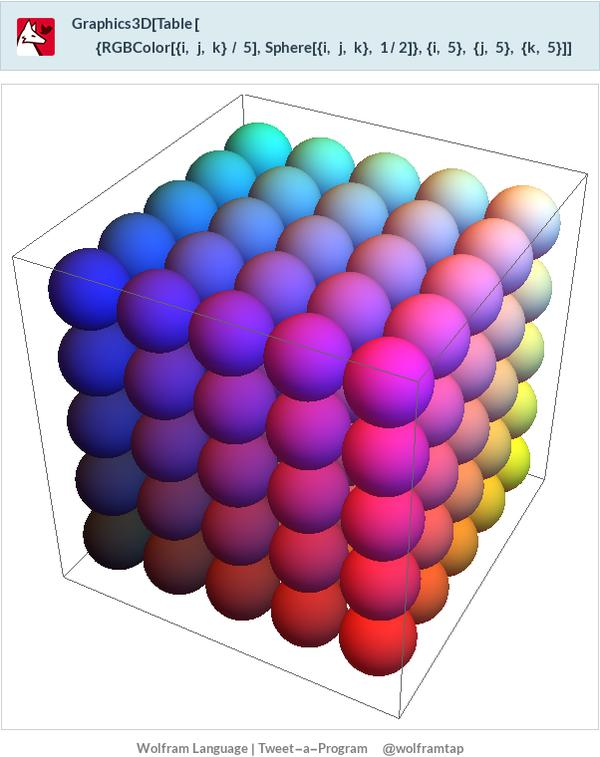# 3D grid like the one in the picture

I am interested on drawing with Sage something like the following pictureThe code that appears in the picture uses Wolfram Language (and I got the picture from this link)

Moreover, what I am interested is to have some function that creates such a picture from a list of points (where by a point I refer to an element of $\mathbb{Z}^3$). Does anybody know how to do this with Sage?

edit retag close merge delete

Sort by » oldest newest most voted

sum(sphere(center=(i,j,k),size=1/2,color=(i/4,j/4,k/4)) for i in range(5) for j in range(5) for k in range(5))

more

Thanks, it is exactly what I wanted. Do you know whether it is easy to write numbers (like a kind of labels) to each one of the balls (in such a way that we can later search for the ball with number 1, for the ball with number 2, etc)